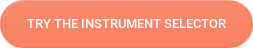Interfacial Tension (IFT) is a measurement of the cohesive or excess energy present at an interface arising from the imbalance of forces between molecules at an interface.

When two different phases (gas/liquid, liquid/liquid, gas/solid or liquid/solid) are in contact with each other the molecules at the interface experience an imbalance of forces. This leads to an accumulation of free energy at the interface. This excess energy is called surface free energy and can be quantified by a measurement of energy/area ratio or the energy required to increase the surface area of the interface by a unit amount. It is also possible to describe this situation as having a line tension or interfacial tension (IFT), which is quantified as a force/length measurement. This force tends to minimize the area of the surface, thus explaining why for example liquid drops and air bubbles are round. The common units for interfacial tension (IFT) are dynes/cm or mN/m. These units are equivalent.Excess energy exists at any type of interface. If one of the phases is the gas phase of a liquid being tested, the measurement is normally referred to as Surface Tension (ST). If the surface investigated is the interface of two immiscible liquids, the measurement is normally referred to as interfacial tension (IFT). In either case, the denser fluid is referred to as the ‘heavy phase’ and the lighter fluid is referred to as the ‘light phase’. Solid surfaces also may be described to have an interfacial tension normally referred to as Surface Free Energy (SFE), but direct measurement of its value is not possible through techniques used for liquids. The Attension® Theta and Attension Sigma series tensiometers provide the means for measuring semi-dynamic and equilibrium interfacial tension.# Let's find the best tensiometer for your measurements!

Our tensiometer selector guides you to your optimal instrument.